# Romberg method

Romberg rule

A method for calculating a definite integral based on Richardson extrapolation. Suppose a valueof some functional is to be calculated; also, let a calculated approximate value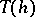depend on a parameterso that as a result of the computations one obtains an approximate equality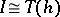. Let some information be known concerning the behaviour of the difference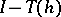as a function of, namely,(1)

whereis a positive integer anddepends on the functional to be approximated, on the function on which this functional is calculated, on the approximating method, and (weakly) on. If simultaneously with,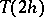is calculated, then by Richardson's method one obtains forthe approximation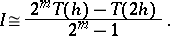(2)

This approximation is the better, the weaker the dependence ofin (1) on. In particular, ifis independent of, then (2) becomes an exact equality.

Romberg's method is used to calculate an integral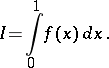The interval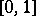is chosen to facilitate the writing; it can be any finite interval, however. Let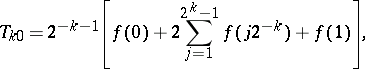(3)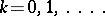Calculations by Romberg's method reduce to writing down the following table:where in the first column one finds the quadrature sums (3) of the trapezium formula. The elements of the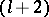-nd column are obtained from the elements of the-st column by the formula(4)

When writing down the table, the main calculating effort is concerned with calculating the elements of the first column. The calculation of the elements of the following columns is a bit more complicated than the calculation of finite differences.

Each element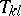in the table is a quadrature sum approximating the integral: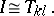(5)

The nodes of the quadrature sumare the points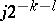,, and its coefficients are positive numbers. The quadrature formula (5) is exact for all polynomials of degree not exceeding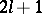.

Under the assumption that the integrand has a continuous derivative onof order, the differencecan be represented in the form (1), where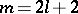. Hence it follows that the elements of the-nd column, calculated by formula (4), are better Richardson approximations than the elements of the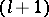-st column. In particular, the following representation is valid for the error of the quadrature trapezium formulaand the Richardson method provides a better approximation to: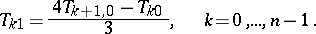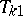turns out to be a quadrature sum of the Simpson formula, and since for the error of this formula the following representation holds:one can again use the Richardson method, etc.

In Romberg's method, to approximateone takes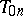; also, one assumes the continuous derivativeonto exist. A tentative idea of the precision of the approximationcan be obtained by comparingto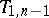.

This method was for the first time described by W. Romberg .